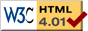An Australian Business Number (ABN) is a single identifier for all business dealings with the tax office and for dealings with other government departments and agencies.

You can use the form below to test if a ABN is valid.

ABN

If you don't have a ABN, you can use the following number to test the system: ABN 53 004 085 616.

## How does it work ?

The verification algorithm embedded in the Australian Business Number is known as a check digit algorithm: one of the digits (the first two in this case) depends upon the others, meaning that if one digit is changed, the last digit will not be correct anymore.

The general procedure is as follows: firstly, subtract 1 from the first digit of the Australian Business Number of interest (our example number 53 004 085 616 becomes 43 004 085 616). Each digit of the new number is multiplied by a given weight (different weights are used for the different digits), and the sum of these products should be a multiple of 89. If it is not the case, there is an error in your number.

Here is a complete example for the number 53 004 085 616. As we said, we substract 1 from the first number on the left, which gives us 43 004 085 616. We multiply each digit of this new number by the weights given below:

 4 3 0 0 4 0 8 5 6 1 6 x x x x x x x x x x x 10 1 3 5 7 9 11 13 15 17 19 = = = = = = = = = = = 40 + 3 + 0 + 0 + 28 + 0 + 88 + 65 + 90 + 17 + 114 = 445

We then calculate the sum 40 + 3 + 0 + 0 + 28 + 0 + 88 + 65 + 90 + 17 + 114 = 445. Since 445 = 89 * 5, it is a multiple of 89, hence the number is valid.

## Background and sources

References:Last modified: Thu Dec 11 19:12:00 EST 2003